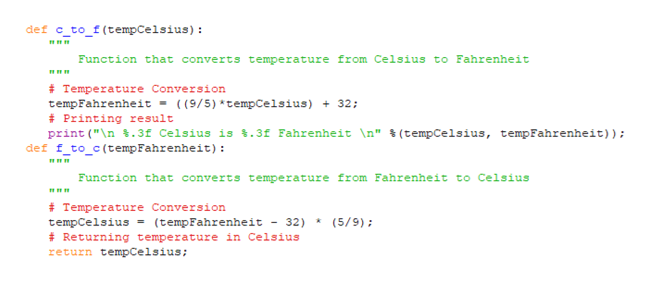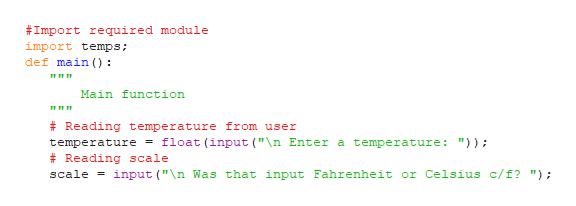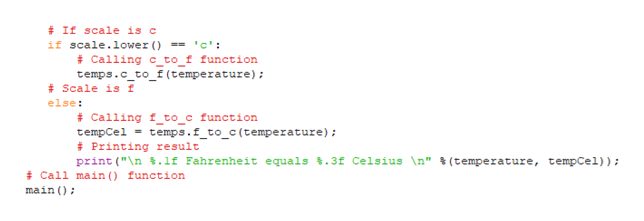2. Write a Python program that can convert a Fahrenheit temperature to Celsius, or vice versa. The program should use two custom functions, f_to_c and c_to_f,  to perform the conversions. Both of these functions should be defined in a custom module named temps. Custom function c_to_f should be a void function defined to take a Celsius temperature as a parameter. It should calculate and print the equivalent Fahrenheit temperature accurate to three decimal places. Custom function f_to_c should be a value-returning function defined to take a Fahrenheit temperature as a parameter. This function should calculate the equivalent Celsius temperature and return it. In the main function, your program should:prompt the user to enter a temperature (as a float type).indicate the temperature scale of the temperature just entered.call the appropriate function from the temps module.if the Celsius temperature is being determined, it should be displayed accurate to three decimal places.EXAMPLE OUTPUT 1Enter a temperature 32Was that input Fahrenheit or Celsius c/f? f32.0 Fahrenheit equals 0.000 CelsiusEXAMPLE OUTPUT 2Enter a temperature 100Was that input Fahrenheit or Celsius c/f? c100.0 Celsius is 212.000 Fahrenheit

Question

2. Write a Python program that can convert a Fahrenheit temperature to Celsius, or vice versa. The program should use two custom functions, f_to_c and c_to_f,  to perform the conversions. Both of these functions should be defined in a custom module named temps. Custom function c_to_f should be a void function defined to take a Celsius temperature as a parameter. It should calculate and print the equivalent Fahrenheit temperature accurate to three decimal places. Custom function f_to_c should be a value-returning function defined to take a Fahrenheit temperature as a parameter. This function should calculate the equivalent Celsius temperature and return it. In the main function, your program should:

• prompt the user to enter a temperature (as a float type).
• indicate the temperature scale of the temperature just entered.
• call the appropriate function from the temps module.
• if the Celsius temperature is being determined, it should be displayed accurate to three decimal places.

EXAMPLE OUTPUT 1

Enter a temperature 32
Was that input Fahrenheit or Celsius c/f? f
32.0 Fahrenheit equals 0.000 Celsius

EXAMPLE OUTPUT 2

Enter a temperature 100
Was that input Fahrenheit or Celsius c/f? c
100.0 Celsius is 212.000 Fahrenheit

Step 1

A Python program that can convert a Fahrenheit temperature to Celsius or vice versa, is given below;

Program:

File name: “temps.py”

def c_to_f(tempCelsius):

"""

Function that converts temperature from Celsius to Fahrenheit

"""

# Temperature Conversion

tempFahrenheit = ((9/5)*tempCelsius) + 32;

# Printing result

print("\n %.3f Celsius is %.3f Fahrenheit \n" %(tempCelsius, tempFahrenheit));

def f_to_c(tempFahrenheit):

"""

Function that converts temperature from Fahrenheit to Celsius

"""

# Temperature Conversion

tempCelsius = (tempFahrenheit - 32) * (5/9);

# Returning temperature in Celsius

return tempCelsius;

File name:”temperature.py”

#Import required module

import temps;

def main():

"""

Main function

"""

# Reading temperature from user

temperature = float(input("\n Enter a temperature: "));

scale = input("\n Was that input Fahrenheit or Celsius c/f? ");

# If scale is c

if scale.lower() == 'c':

# Calling c_to_f function

temps.c_to_f(temperature);

# Scale is f

else:

# Calling f_to_c function

tempCel = temps.f_to_c(temperature);

# Printing result

print("\n %.1f Fahrenheit equals %.3f Celsius \n" %(temperature, tempCel));

# Call main() function

main();

Screenshot of program “temps.py”:help_outlineImage Transcriptionclosedef c_to_f(tempCelsius) Function that converts temperature from Celsius to Fahrenheit # Temperature Conversion tempFahrenheit - ( (9/5)*tempCelsius)32; Printing result print ("\n .3f Celsius is .3f Fahrenheit \n" % (tempCelsius, tempFahrenheit)); def f_to_c(temp Fahrenheit) Function that converts temperature from Fahrenheit to Celsius # Temperature Conversion tempCelsius Returning temperature in Celsius return tempCelsius (5/9) (tempFahrenheit - 32) fullscreen
Step 2

Screenshot of program “temperature.py” #1:help_outlineImage Transcriptionclose#Import required module import temps; def main () Main function # Reading temperature from user float (input ("\n Enter a temperature: ")); temperature Reading scale input ("\n Was that input Fahrenheit or Celsius c/f? "); scale fullscreen
Step 3

Screenshot of program “temperatu...help_outlineImage Transcriptionclose#If scale is c if scale.lower () = 'c': #Calling c to f function temps.c_to_f (temperature) Scale is f else Calling f_to_c function tempCel temps.f_to_c(temperature) Printing result print ("n.lf Fahrenheit equals %.3f Celsius \n" % (temperature, tempCel)); #Call main function main fullscreen

Want to see the full answer?

See Solution

Want to see this answer and more?

Our solutions are written by experts, many with advanced degrees, and available 24/7

See Solution
Tagged in

Python